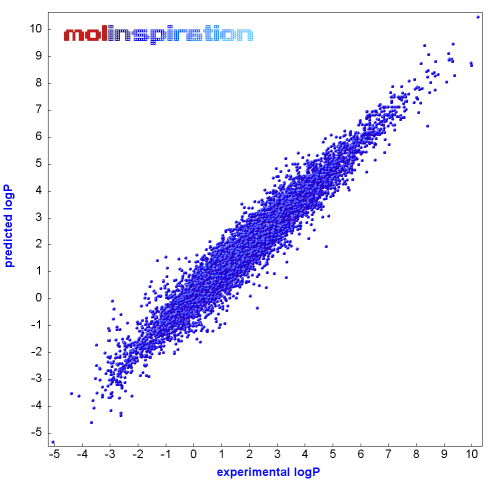# Question 56dce

Apr 11, 2015

You can't calculate the $\text{p"K_"a}$ or $\text{p"K_"b}$ of an unknown drug if you know nothing about it.

But the $\text{p"K_"a}$ and $\text{p"K_"b}$ values are important, because they determine how much of the drug is in the ionic and uncharged forms at a given pH.

You can use the Henderson-Hasselbalch Equation to calculate that

"% ionized" = "100 %"/( 1 + 10^(q("pH" –"p"K_"a"))#

where the charge $q$ = +1 for an acid and -1 for a base.

When $\text{pH"= "p"K_"a}$, 50% of the drug is ionized and 50% is uncharged.

• The ionized form is water-soluble and cannot cross a membrane.

• The uncharged form is lipid-soluble and cannot cross a membrane

When you design a new drug, you can't often predict its $\text{p"K_"a}$, but you can make a good guess about its solubility.

Chemists use the partition ratios to measure relative solubilities in two solvents.

The partition ratio $P$ is the ratio of the concentrations of the unionized drug in two immiscible phases, such as water and octan-1-ol.

${P}_{\text{o/w}} = \frac{{\left[S\right]}_{o}}{{\left[S\right]}_{w}}$

where ${P}_{\text{o/w}}$ is the partition ratio between octanol and water, and $\left[S\right]$ represents the solubility of the drug in the two solvents.

This is usually expressed in logarithmic form:

$\log P = \log \left(\frac{{\left[S\right]}_{o}}{{\left[S\right]}_{w}}\right)$

$\log P$ is a measure of the lipophilicity of the drug.

Chemists have devised computer programs from a database of up to 12 000 compounds and over 200 types of molecular "fragments" to predict $\log P$ values.The programs can predict $\log P$ within ±0.5 units 80 % of the time.